• lstm时间序列预测python
千次阅读
2022-02-05 08:27:22

## 时间序列预测 | Python实现LSTM多变量时间序列数据预测

### 基本介绍

时间序列分析（Time-Series Analysis）是指将原来的销售分解为四部分来看——趋势、周期、时期和不稳定因素，然后综合这些因素，提出销售预测。强调的是通过对一个区域进行一定时间段内的连续遥感观测，提取图像有关特征，并分析其变化过程与发展规模。当然，首先需要根据检测对象的时相变化特点来确定遥感监测的周期，从而选择合适的遥感数据。
时间序列分析：时间序列表示基于时间顺序的一系列数据。它可以是秒、分钟、小时、天、周、月、年。未来的数据将取决于它以前的值。
在现实世界的案例中，我们主要有两种类型的时间序列分析：单变量时间序列、多元时间序列，对于单变量时间序列数据，我们将使用单列进行预测。单变量时间序列只有一列，因此即将到来的未来值将仅取决于它之前的值。但是在多元时间序列数据的情况下，将有不同类型的特征值并且目标数据将依赖于这些特征。

• 在执行多元时间序列分析时必须记住一件事，我们需要使用多个特征预测当前的目标。
• 在训练时，如果我们使用 5 列 [feature1, flstm python 深度学习 时序模型
更多相关内容
• CNN结合BiLSTM实现数据预测python代码.zipmatlab
• 主要介绍了Python中利用LSTM模型进行时间序列预测分析的实现，文中通过示例代码介绍的非常详细，对大家的学习或者工作具有一定的参考学习价值，需要的朋友们下面随着小编来一起学习学习吧
• GA-LSTM 遗传算法优化的lstm 预测代码 python实现 直接就可以运行 GA.PY 具体可以通过lstm.py的load数据进行修改
• 时间序列预测问题中，建立LSTM模型，采用python语言代码实现
• 1.BP神经网络预测python） 2.mlp多层感知机预测python） 下边是基于Python的简单的BP神经网络预测，多输入多输出，下边是我的数据，红色部分预测蓝色 2,3行输入，第4行输出 3,4行输入，第5行输出 …以此类推 ...

可以参考新发布的文章
1.BP神经网络预测（python）
2.mlp多层感知机预测（python）
下边是基于Python的简单的BP神经网络预测，多输入多输出，下边是我的数据，红色部分预测蓝色
2,3行输入，第4行输出
3,4行输入，第5行输出
…以此类推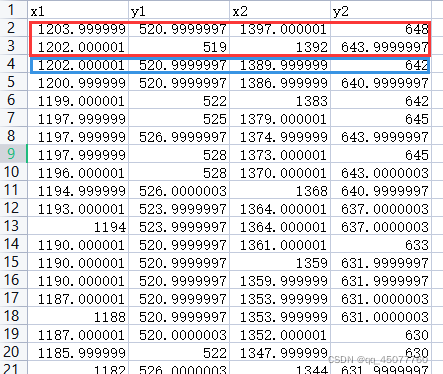简单利索，直接上代码

import matplotlib
import numpy as np
import matplotlib.pyplot as plt
import pandas as pd
import sklearn.metrics
from IPython.core.display import SVG
from keras.layers import LSTM, Dense, Bidirectional
from keras.losses import mean_squared_error
from keras.models import Sequential
from keras.utils.vis_utils import model_to_dot, plot_model
from matplotlib import ticker
from keras import regularizers
from pandas import DataFrame, concat
from sklearn import metrics
# from sklearn.impute import SimpleImputer
from sklearn.model_selection import train_test_split
from sklearn.preprocessing import StandardScaler
from sklearn.preprocessing import MinMaxScaler
#from statsmodels.tsa.seasonal import seasonal_decompose
import tensorflow as tf
import seaborn as sns
import os
os.environ['TF_CPP_MIN_LOG_LEVEL'] = '2'
matplotlib.rcParams['font.sans-serif'] = ['SimHei']
matplotlib.rcParams['axes.unicode_minus'] = False

# 把object类型转换为float
data_origin = DataFrame(data_origin, dtype=float)
# 打印显示所有列
pd.set_option('display.max_columns', None)
# 获得数据的描述信息
# print(data_dropDate.describe())
return data_origin

#单维最大最小归一化和反归一化函数
# 对于训练数据进行归一化之后。使用训练数据的最大最小值（训练数据的范围）对于测试数据进行归一化 保留周期和范围信息
def Normalize(df,low,high):
delta = high - low
for i in range(0,df.shape):
for j in range(0,df.shape):
df[i][j] = (df[i][j]-low[j])/delta[j]
return df
# 定义反归一化函数
def FNormalize(df,low,high):
delta = high - low
for i in range(0,df.shape):
for j in range(0,df.shape):
df[i][j] = df[i][j] * delta[j] + low[j]
return df

# 数据集转换成监督学习问题
# 数据集转换成监督学习函数
def series_to_supervise(data,n_in=1,n_out=1,dropnan=True):
'''该函数有四个参数：

data：作为列表或 2D NumPy 数组的观察序列。必需的。
n_in ：作为输入 ( X )的滞后观察数。值可能在 [1..len(data)] 之间可选。默认为 1。
n_out：作为输出的观察数（y）。值可能在 [0..len(data)-1] 之间。可选的。默认为 1。
dropnan：布尔值是否删除具有 NaN 值的行。可选的。默认为真。
该函数返回一个值：

return：用于监督学习的系列 Pandas DataFrame。'''
n_vars = 1 if type(data) is list else data.shape
df = DataFrame(data)
cols , names = list(),list()
# input sequence(t-n,...,t-1)
for i in range(n_in,0,-1):
cols.append(df.shift(i))
names += [('var%d(t-%d)' %(j+1,i)) for j in range(n_vars)]
# forecast sequence (t, t+1, ... t+n)
for i in range(0, n_out):
cols.append(df.shift(-i))
if i == 0:
names += [('var%d(t)' % (j+1)) for j in range(n_vars)]
else:
names += [('var%d(t+%d)' % (j+1, i)) for j in range(n_vars)]
# put it all together
agg = concat(cols, axis=1)
agg.columns = names
# drop rows with NaN values
if dropnan:
agg.dropna(inplace=True)
return agg
# 定义一个名为split_sequences()的函数，它将采用我们定义的数据集，其中包含时间步长的行和并行序列的列，并返回输入/输出样本。
# split a multivariate sequence into samples
def split_sequences(sequences, n_steps):
X, y = list(), list()
for i in range(len(sequences)):
# # find the end of this pattern
end_ix = i + n_steps
if end_ix > len(sequences)-n_steps:
break
# gather input and output parts of the pattern
seq_x,seq_y = sequences[i:end_ix,:-1],sequences[end_ix,:-1]
X.append(seq_x)
y.append(seq_y)
return np.array(X),np.array(y)

path = 'E:/YOLO/yolov5-master/digui/1/origin.xlsx'
print(data_origin.shape)

# 先将数据集分成训练集和测试集
train_Standard = data_origin.iloc[:60,:]  # （145,23）,前60行
test_Standard = data_origin.iloc[60:,:]  # （38,23），后边

# 再转换为监督学习问题
n_in = 2
n_out = 1
train_Standard_supervise = series_to_supervise(train_Standard,n_in,n_out)  # (144,46)
test_Standard_supervise = series_to_supervise(test_Standard,n_in,n_out)  # (37,46)
print('test_Standard_supervise')

# 将训练集和测试集分别分成输入和输出变量。最后，将输入（X）重构为 LSTM 预期的 3D 格式，即 [样本，时间步，特征]
# split into input and output
train_Standard_supervise = train_Standard_supervise.values
test_Standard_supervise = test_Standard_supervise.values
train_X,train_y = train_Standard_supervise[:,:8],train_Standard_supervise[:,8:]
test_X,test_y = test_Standard_supervise[:,:8],test_Standard_supervise[:,8:]

#归一化
scaler = StandardScaler()
train_X=scaler.fit_transform(train_X)
train_y = train_y.reshape(train_y.shape,4)
train_y=scaler.fit_transform(train_y)
test_X=scaler.fit_transform(test_X)
test_y = test_y.reshape(test_y.shape,4)
test_y=scaler.fit_transform(test_y)

# reshape input to be 3D [samples,timeseps,features]
train_X = train_X.reshape((train_X.shape,1,train_X.shape))
test_X = test_X.reshape((test_X.shape,1,test_X.shape))
print(train_X.shape,train_y.shape,test_X.shape,test_y.shape)  #(145, 1, 22) (145,) (38, 1, 22) (38,)
groups = 
i = 1
plt.figure()
for group in groups:
# 定义模型
model = Sequential()
# 输入层维度：input_shape（步长，特征数）
# 拟合模型
history = model.fit(train_X, train_y, epochs=100, batch_size=group, validation_data=(test_X, test_y))

plt.subplot(len(groups),1,i)
plt.plot(history.history['loss'], label='train')
plt.plot(history.history['val_loss'], label='test')
plt.xlabel('epoch')
plt.ylabel('loss')
plt.title('batch_size = %d' %group)
plt.legend()
i += 1
plt.show()

# 预测测试集
yhat = model.predict(test_X)
test_X = test_X.reshape((test_X.shape, test_X.shape))
# invert scaling for forecast
# invert scaling for actual
test_y = test_y.reshape((len(test_y), 4))
yhat=scaler.inverse_transform(yhat)
test_y=scaler.inverse_transform(test_y)
# calculate RMSE
#print(test_y)
#print(yhat)

rmse = np.sqrt(mean_squared_error(test_y, yhat))
mape = np.mean(np.abs((yhat-test_y)/test_y))*100
print('=============rmse==============')
print(rmse)
print('=============mape==============')
print(mape,'%')
print("R2 = ",metrics.r2_score(test_y, yhat)) # R2
# 画出真实数据和预测数据的对比曲线图
plt.plot(test_y,color = 'red',label = 'true')
plt.plot(yhat,color = 'blue', label = 'pred')
plt.title('Prediction')
plt.xlabel('Time')
plt.ylabel('value')
plt.legend()
plt.show()



这是出来的训练集和测试集损失图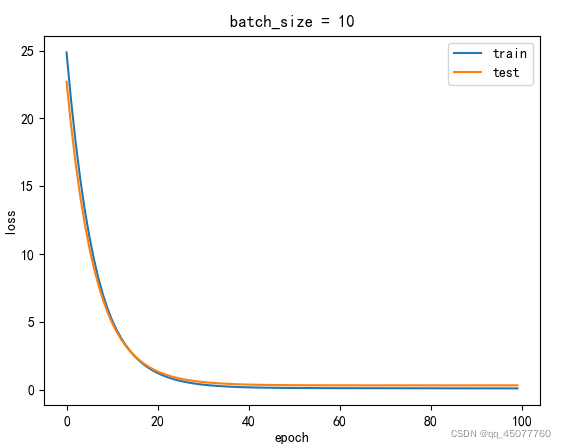下边是预测值和真实值的结果图，其中
mape=0.15912692740815412 %
R2 = 0.9762727107502187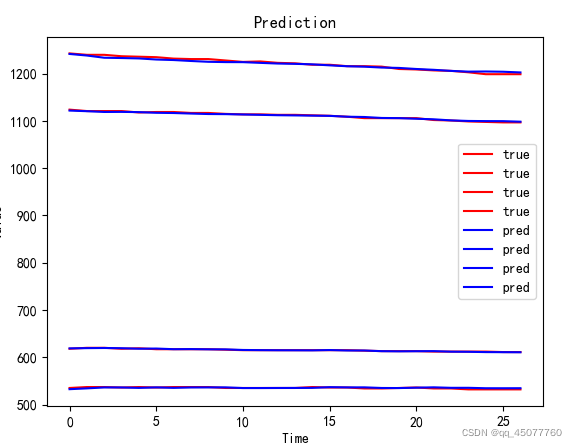GRU的修改

    model = Sequential()
# 输入层维度：input_shape（步长，特征数）
# 拟合模型
history = model.fit(train_X, train_y, epochs=100, batch_size=group, validation_data=(test_X, test_y))


改成

model = Sequential()
activation='relu'
)
)
activation='sigmoid'
))
model.compile(loss='mae',optimizer='rmsprop')
# 拟合模型
batchsize = 10
history = model.fit(train_X, train_y, epochs=100, batch_size=batchsize, validation_data=(test_X, test_y))


剩下的就剩调参了

展开全文python lstm gru
• 目录LSTM神经网络LSTM原理LSTM数据下载LSTM程序设计导入Python包时序数据处理LSTM模块定义模型训练模型评估参数设定运行结果参考资料致谢 LSTM神经网络 长短期记忆网络——通常简称为“LSTM”——是一种特殊的 RNN...

### LSTM神经网络

长短期记忆网络——通常简称为“LSTM”——是一种特殊的 RNN，能够学习长期依赖关系。它们由Hochreiter & Schmidhuber (1997)引入，并在后续工作中被许多人提炼和推广。1它们在处理各种各样的问题时效果非常好，现在被广泛使用。LSTM 被明确设计为避免长期依赖问题。长时间记住信息实际上是他们的默认行为，而不是他们努力学习的东西！所有循环神经网络都具有神经网络重复模块链的形式。在标准 RNN 中，这个重复模块将具有非常简单的结构，例如单个 tanh 层。

展开全文神经网络 深度学习 python 机器学习
• LSTM时间序列预测 python代码——import pandas as pd import numpy as np import matplotlib.pyplot as plt import tensorflow as tf tf.reset_default_graph() #——————————————————导入数据...
• LSTM构建使用Keras Python包来预测时间序列步骤和序列。 包括正弦波和股票市场数据
• 本代码采用python语言写的一个LSTM时间序列预测销量LSTM 时间序列
• 今天给大家分享一波使用 LSTM 进行端到端时间序列预测的完整代码和详细解释。我们先来了解两个主题：什么是时间序列分析？什么是 LSTM时间序列分析：时间序列表示基于时间顺序的一系列数据。它可以是秒、分钟、...

来源：DeepHub IMBA

大家经常会遇到一些需要预测的场景，比如预测品牌销售额，预测产品销量。

今天给大家分享一波使用 LSTM 进行端到端时间序列预测的完整代码和详细解释。

我们先来了解两个主题：

• 什么是时间序列分析？

• 什么是 LSTM？

时间序列分析：时间序列表示基于时间顺序的一系列数据。它可以是秒、分钟、小时、天、周、月、年。未来的数据将取决于它以前的值。

在现实世界的案例中，我们主要有两种类型的时间序列分析：

• 单变量时间序列

• 多元时间序列

对于单变量时间序列数据，我们将使用单列进行预测。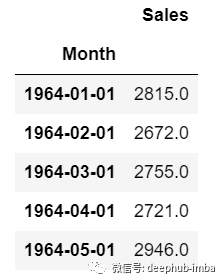正如我们所见，只有一列，因此即将到来的未来值将仅取决于它之前的值。

但是在多元时间序列数据的情况下，将有不同类型的特征值并且目标数据将依赖于这些特征。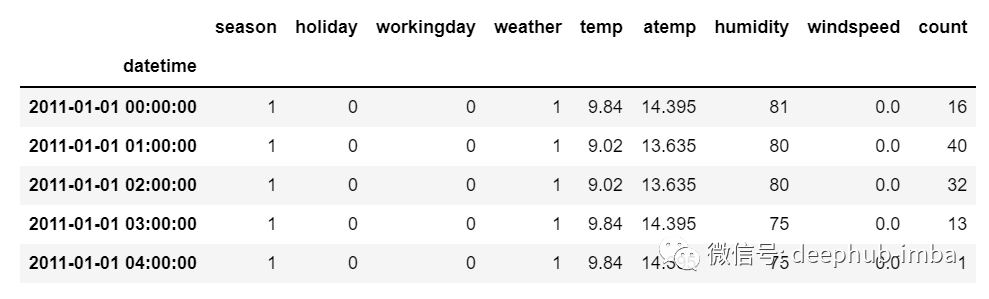正如在图片中看到的，在多元变量中将有多个列来对目标值进行预测。（上图中“count”为目标值）

在上面的数据中，count不仅取决于它以前的值，还取决于其他特征。因此，要预测即将到来的count值，我们必须考虑包括目标列在内的所有列来对目标值进行预测。

在执行多元时间序列分析时必须记住一件事，我们需要使用多个特征预测当前的目标，让我们通过一个例子来理解：

在训练时，如果我们使用 5 列 [feature1, feature2, feature3, feature4, target] 来训练模型，我们需要为即将到来的预测日提供 4 列 [feature1, feature2, feature3, feature4]。

LSTM

本文中不打算详细讨论LSTM。所以只提供一些简单的描述，如果你对LSTM没有太多的了解，可以参考我们以前发布的文章。

LSTM基本上是一个循环神经网络，能够处理长期依赖关系。

假设你在看一部电影。所以当电影中发生任何情况时，你都已经知道之前发生了什么，并且可以理解因为过去发生的事情所以才会有新的情况发生。RNN也是以同样的方式工作，它们记住过去的信息并使用它来处理当前的输入。RNN的问题是，由于渐变消失，它们不能记住长期依赖关系。因此为了避免长期依赖问题设计了lstm。

现在我们讨论了时间序列预测和LSTM理论部分。让我们开始编码。

让我们首先导入进行预测所需的库：

import numpy as np
import pandas as pd
from matplotlib import pyplot as plt
from tensorflow.keras.models import Sequential
from tensorflow.keras.layers import LSTM
from tensorflow.keras.layers import Dense, Dropout
from sklearn.preprocessing import MinMaxScaler
from keras.wrappers.scikit_learn import KerasRegressor
from sklearn.model_selection import GridSearchCV

加载数据，并检查输出：

df=pd.read_csv("train.csv",parse_dates=["Date"],index_col=)
df.head()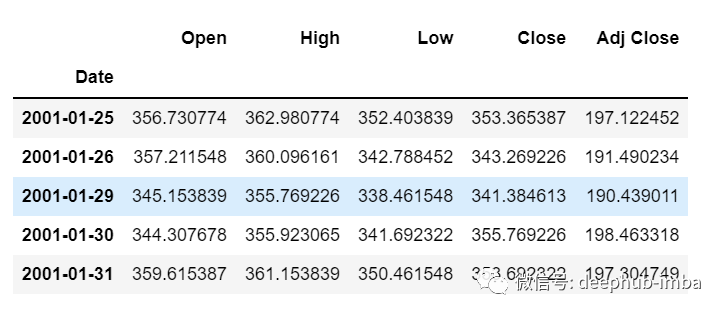df.tail()

现在让我们花点时间看看数据：csv文件中包含了谷歌从2001-01-25到2021-09-29的股票数据，数据是按照天数频率的。

[如果您愿意，您可以将频率转换为“B”[工作日]或“D”，因为我们不会使用日期，我只是保持它的现状。]

这里我们试图预测“Open”列的未来值，因此“Open”是这里的目标列。

让我们看一下数据的形状：

df.shape
(5203,5)

现在让我们进行训练测试拆分。这里我们不能打乱数据，因为在时间序列中必须是顺序的。

test_split=round(len(df)*0.20)
df_for_training=df[:-1041]
df_for_testing=df[-1041:]
print(df_for_training.shape)
print(df_for_testing.shape)

(4162, 5)
(1041, 5)

可以注意到数据范围非常大，并且它们没有在相同的范围内缩放，因此为了避免预测错误，让我们先使用MinMaxScaler缩放数据。(也可以使用StandardScaler)

scaler = MinMaxScaler(feature_range=(0,1))
df_for_training_scaled = scaler.fit_transform(df_for_training)
df_for_testing_scaled=scaler.transform(df_for_testing)
df_for_training_scaled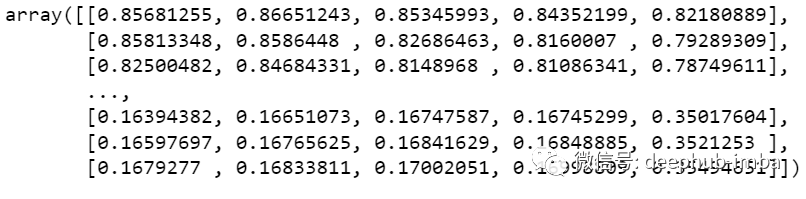将数据拆分为X和Y，这是最重要的部分，正确阅读每一个步骤。

def createXY(dataset,n_past):
dataX = []
dataY = []
for i in range(n_past, len(dataset)):
dataX.append(dataset[i - n_past:i, 0:dataset.shape])
dataY.append(dataset[i,0])
return np.array(dataX),np.array(dataY)

trainX,trainY=createXY(df_for_training_scaled,30)
testX,testY=createXY(df_for_testing_scaled,30)

让我们看看上面的代码中做了什么：

N_past是我们在预测下一个目标值时将在过去查看的步骤数。

这里使用30，意味着将使用过去的30个值(包括目标列在内的所有特性)来预测第31个目标值。

因此，在trainX中我们会有所有的特征值，而在trainY中我们只有目标值。

让我们分解for循环的每一部分：

对于训练，dataset = df_for_training_scaled, n_past=30

当i= 30：

data_X.addend (df_for_training_scaled[i - n_past:i, 0:df_for_training.shape])

从n_past开始的范围是30，所以第一次数据范围将是-[30 - 30,30,0:5] 相当于 [0:30,0:5]

因此在dataX列表中，df_for_training_scaled[0:30,0:5]数组将第一次出现。

现在, dataY.append(df_for_training_scaled[i,0])

i = 30，所以它将只取第30行开始的open(因为在预测中，我们只需要open列，所以列范围仅为0，表示open列)。

第一次在dataY列表中存储df_for_training_scaled[30,0]值。

所以包含5列的前30行存储在dataX中，只有open列的第31行存储在dataY中。然后我们将dataX和dataY列表转换为数组，它们以数组格式在LSTM中进行训练。

我们来看看形状。

print("trainX Shape-- ",trainX.shape)
print("trainY Shape-- ",trainY.shape)

(4132, 30, 5)
(4132,)

print("testX Shape-- ",testX.shape)
print("testY Shape-- ",testY.shape)

(1011, 30, 5)
(1011,)

4132 是 trainX 中可用的数组总数，每个数组共有 30 行和 5 列， 在每个数组的 trainY 中，我们都有下一个目标值来训练模型。

让我们看一下包含来自 trainX 的 (30,5) 数据的数组之一 和 trainX 数组的 trainY 值：

print("trainX-- \n",trainX)
print("trainY-- ",trainY)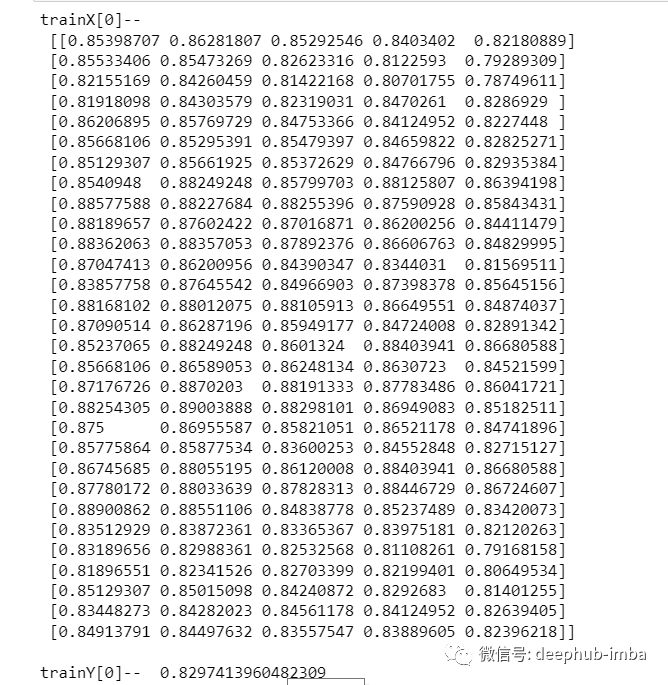如果查看 trainX 值，会发现到它与 trainX 中的数据相同（第一列除外），因为我们将看到前 30 个来预测第 31 列，在第一次预测之后它会自动移动 到第 2 列并取下一个 30 值来预测下一个目标值。

让我们用一种简单的格式来解释这一切：

trainX — — →trainY

[0 : 30,0:5] → [30,0]

[1:31, 0:5] → [31,0]

[2:32,0:5] →[32,0]

像这样，每个数据都将保存在 trainX 和 trainY 中。

现在让我们训练模型，我使用 girdsearchCV 进行一些超参数调整以找到基础模型。

def build_model(optimizer):
grid_model = Sequential()

grid_model.compile(loss = 'mse',optimizer = optimizer)
return grid_modelgrid_model = KerasRegressor(build_fn=build_model,verbose=1,validation_data=(testX,testY))

parameters = {'batch_size' : [16,20],
'epochs' : [8,10],

grid_search = GridSearchCV(estimator = grid_model,
param_grid = parameters,
cv = 2)

如果你想为你的模型做更多的超参数调整，也可以添加更多的层。但是如果数据集非常大建议增加 LSTM 模型中的时期和单位。

在第一个 LSTM 层中看到输入形状为 (30,5)。它来自 trainX 形状。

(trainX.shape,trainX.shape) → (30,5)

现在让我们将模型拟合到 trainX 和 trainY 数据中。

grid_search = grid_search.fit(trainX,trainY)

由于进行了超参数搜索，所以这将需要一些时间来运行。

你可以看到损失会像这样减少：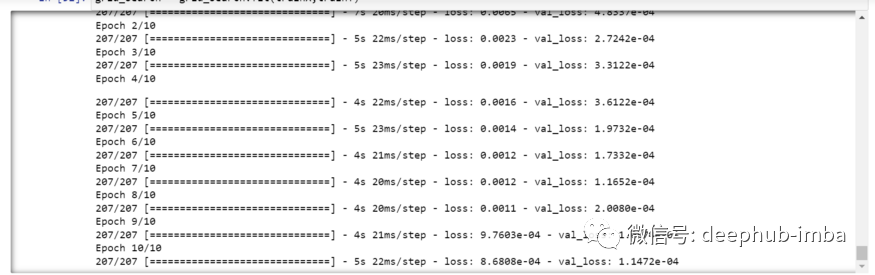现在让我们检查模型的最佳参数。

grid_search.best_params_

{‘batch_size’: 20, ‘epochs’: 10, ‘optimizer’: ‘adam’}

将最佳模型保存在 my_model 变量中。

my_model=grid_search.best_estimator_.model

现在可以用测试数据集测试模型。

prediction=my_model.predict(testX)
print("prediction\n", prediction)
print("\nPrediction Shape-",prediction.shape)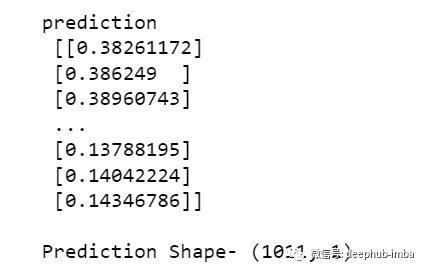testY 和 prediction 的长度是一样的。现在可以将 testY 与预测进行比较。

但是我们一开始就对数据进行了缩放，所以首先我们必须做一些逆缩放过程。

scaler.inverse_transform(prediction)

报错了，这是因为在缩放数据时，我们每行有 5 列，现在我们只有 1 列是目标列。

所以我们必须改变形状来使用 inverse_transform：

prediction_copies_array = np.repeat(prediction,5, axis=-1)

5 列值是相似的，它只是将单个预测列复制了 4 次。所以现在我们有 5 列相同的值 。

prediction_copies_array.shape
(1011,5)

这样就可以使用 inverse_transform 函数。

pred=scaler.inverse_transform(np.reshape(prediction_copies_array,(len(prediction),5)))[:,0]

但是逆变换后的第一列是我们需要的，所以我们在最后使用了 → [:,0]。

现在将这个 pred 值与 testY 进行比较，但是 testY 也是按比例缩放的，也需要使用与上述相同的代码进行逆变换。

original_copies_array = np.repeat(testY,5, axis=-1)
original=scaler.inverse_transform(np.reshape(original_copies_array,(len(testY),5)))[:,0]

现在让我们看一下预测值和原始值：

print("Pred Values-- " ,pred)
print("\nOriginal Values-- " ,original)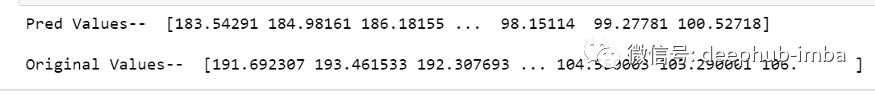最后绘制一个图来对比我们的 pred 和原始数据。

plt.plot(original, color = 'red', label = 'Real Stock Price')
plt.plot(pred, color = 'blue', label = 'Predicted Stock Price')
plt.title('Stock Price Prediction')
plt.xlabel('Time')
plt.legend()
plt.show()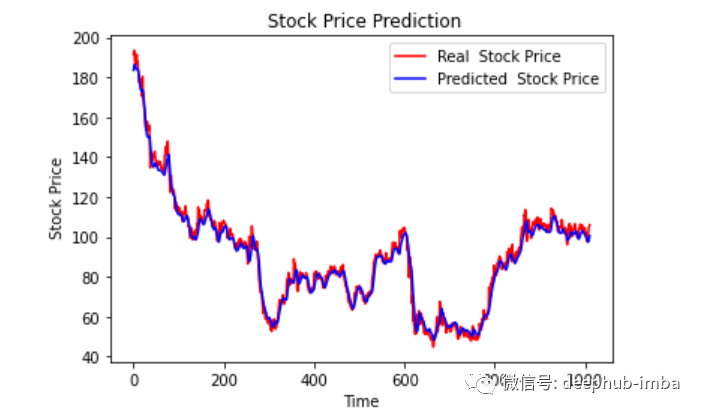看样子还不错，到目前为止，我们训练了模型并用测试值检查了该模型。现在让我们预测一些未来值。

从主 df 数据集中获取我们在开始时加载的最后 30 个值[为什么是 30？因为这是我们想要的过去值的数量，来预测第 31 个值]

df_30_days_past=df.iloc[-30:,:]
df_30_days_past.tail()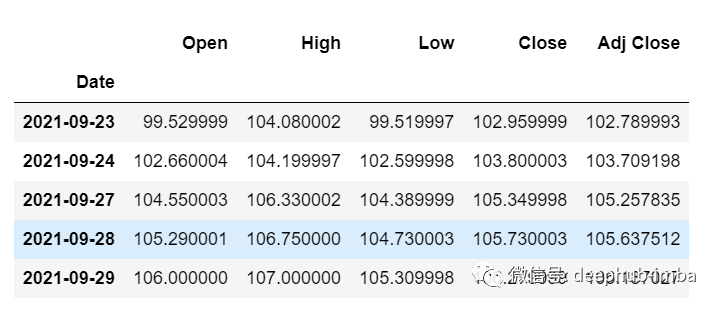可以看到有包括目标列（“Open”）在内的所有列。现在让我们预测未来的 30 个值。

在多元时间序列预测中，需要通过使用不同的特征来预测单列，所以在进行预测时我们需要使用特征值（目标列除外）来进行即将到来的预测。

df_30_days_future=pd.read_csv("test.csv",parse_dates=["Date"],index_col=)
df_30_days_future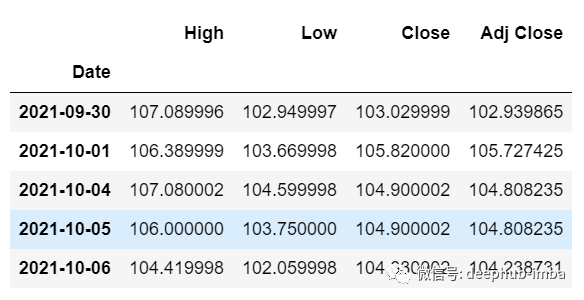剔除“Open”列后，使用模型进行预测之前还需要做以下的操作：

缩放数据，因为删除了‘Open’列，在缩放它之前，添加一个所有值都为“0”的Open列。

缩放后，将未来数据中的“Open”列值替换为“nan”

现在附加 30 天旧值和 30 天新值（其中最后 30 个“打开”值是 nan）

df_30_days_future["Open"]=0
old_scaled_array=scaler.transform(df_30_days_past)
new_scaled_array=scaler.transform(df_30_days_future)
new_scaled_df=pd.DataFrame(new_scaled_array)
new_scaled_df.iloc[:,0]=np.nan
full_df=pd.concat([pd.DataFrame(old_scaled_array),new_scaled_df]).reset_index().drop(["index"],axis=1)

full_df  形状是 (60,5)，最后第一列有 30 个 nan 值。

要进行预测必须再次使用 for 循环，我们在拆分 trainX 和 trainY 中的数据时所做的。但是这次我们只有 X，没有 Y 值。

full_df_scaled_array=full_df.values
all_data=[]
time_step=30
for i in range(time_step,len(full_df_scaled_array)):
data_x=[]
data_x.append(
full_df_scaled_array[i-time_step :i , 0:full_df_scaled_array.shape])
data_x=np.array(data_x)
prediction=my_model.predict(data_x)
all_data.append(prediction)
full_df.iloc[i,0]=prediction

对于第一个预测，有之前的 30 个值，当 for 循环第一次运行时它会检查前 30 个值并预测第 31 个“Open”数据。

当第二个 for 循环将尝试运行时，它将跳过第一行并尝试获取下 30 个值 [1:31] 。这里会报错错误因为Open列最后一行是 “nan”，所以需要每次都用预测替换“nan”。

最后还需要对预测进行逆变换：

new_array=np.array(all_data)
new_array=new_array.reshape(-1,1)
prediction_copies_array = np.repeat(new_array,5, axis=-1)
y_pred_future_30_days = scaler.inverse_transform(np.reshape(prediction_copies_array,(len(new_array),5)))[:,0]
print(y_pred_future_30_days)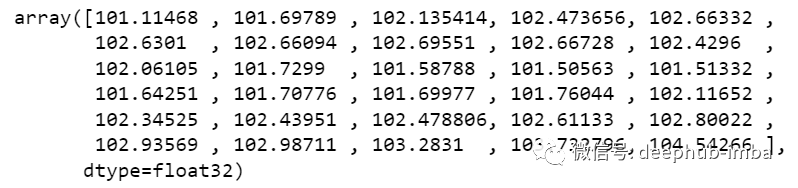这样一个完整的流程就已经跑通了。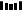●分析师如何正确的提建议？
●品牌知名度分析
展开全文python 机器学习 人工智能 深度学习 java
• LSTM Time Series Prediction with Bayesian optimization.zipLSTM
• 时间序列预测讲义（ARIMA&LSTM;）及python代码，首先讲述了基本概念及公式，然后提供了python代码时间序列
• TensorFlow框架下的LSTM时间序列预测【简易】tensorflow lstm python
• ## pytorch LSTM时间序列预测

千次阅读 热门讨论 2021-12-02 11:28:49pytorch lstm python
• 如何使用LSTM预测成交量加权平均价格，这是一个既有挑战性又很有趣的问题。本文将使用PyTorch训练有关比特币交易数据的Long Short Term Memory Neural Network(LSTM， 长短记忆神经网络)，并使用它预测看不见的交易...
• 　关于理论部分，可以参考这两篇文章（RNN、LSTM），本文主要从数据、代码角度，利用LSTM进行时间序列预测。（1）原始时间序列数据（只列出了18行）1455.219971 1399.420044 1402.109985 1403.449951 1441.469971 ...python LSTM 时间序列预测
• ## LSTM时间序列预测

千次阅读 2021-05-23 09:19:36
LSTM时间序列预测（单维，多步预测一步） 文章目录LSTM时间序列预测（单维，多步预测一步）前言使用步骤1.引入库2.设置一下，画图时可以显示汉字3.设置监督学习格式3.读取数据4.缩放数据：归一化5.划分训练集和测试...机器学习 python lstm
• 单变量多变量预测小例子，时间序列转换成稳定数据，时间序列转换成监督数据
• 数据是单维一例的，基于时间节点的预测，matlab出图，程序包含LSTM程序单独运行、SSA-LSTM程序运行、两种程序对比。前70%训练，后面30%预测，大约有2000个数据，代码有详细说明，可供调整学习。
• # 代码功能: LS-TM 循环神经网络，预测时间序列---------------------------- # 第1步: 处理原始数据集, 归一化，制作X_train、Y_train、X_test、Y_test # 第2步: 训练LS-TM网络, epoch=300 ...# 第3步: 时间序列预测
• # -*- coding: utf-8 -*-importnumpyasnpimportnumpyimportmatplotlib.pyplotaspltimportpandasimportmathfromkeras.modelsimportSequentialfromkeras.layersimportDense,LSTM,Dropoutfromsklearn.preprocessingimpo...
• 实现隧道变形时间序列预测，一个相关影响变量，实现变形预测
• ## LSTM时间序列预测

千次阅读 2020-12-09 21:41:54
我们使用它来进行LSTM时间序列预测的实验。数据如图所示第一列为时间 第二列为数据编写代码头文件 import numpy import matplotlib.pyplot as plt from keras.models import Sequential from keras.layers impo
• 1 keras 1.1 入门 资源： ...seid=13437359564386162135 kera是一种深度学习框架，用起来比tensorflow简单 ...安装：安装好python环境后，要安装tensorflow（有CPU和GPU版本，为了简单可以安装CPU版本），之后lstm tensorflow 深度学习
• tensorflow下用LSTM网络进行时间序列预测，实时多变量预测以及对于未来数据的单变量预测。
• 时间序列预测 | Python实现CNN-LSM模型时间序列预测 目录时间序列预测 | Python实现CNN-LSM模型时间序列预测基本介绍环境准备程序设计数据处理模型搭建结果分析模型评估总结 基本介绍 这篇文章将带大家通过...python 深度学习 lstm...